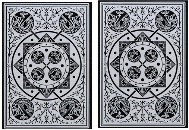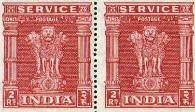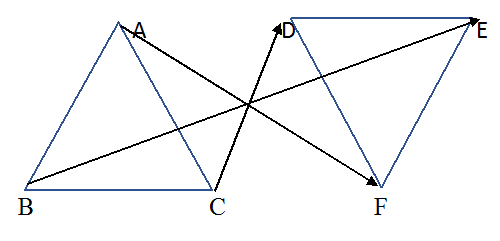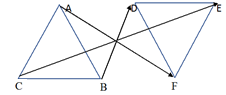NCERT Class 7 Maths Congruence of Triangles

# NCERT Class 7 Maths Congruence of Triangles

This chapter begins with an introduction to Congruence of Triangles by explaining the basic concept of congruence among plane figures,line segments, angles and finally triangles.The congruence of triangles is the main topic of discussion and hence it is explained elaborately.Then the criteria for congruence of triangles such as SSS Congruence Criterion,SAS Congruence Criterion, ASA Congruence Criterion, are explained through SSS game, SAS game and ASA games respectively. Finally, congruence among right angled triangles i.e. RHS Congruence Criterion is explained in detail.

## Chapter 7 Ex.7.1 Question 1

Complete the following statements:

a) Two-line segments are congruent if ___________.

b) Among two congruent angles, one has a measure of $$70^\circ$$; the measure of the other angle is ___________.

c) When we write $$∠A = ∠B,$$ we actually mean ___________.

### Solution

Steps:

a) they have same length.

b) $$70^\circ$$

c) $$m ∠A = m ∠B$$ (m stands for measure of the angle)

## Chapter 7 Ex.7.1 Question 2

Give any two real-life examples for congruent shapes.

### Solution

Steps:

a) Cards of same playing card deck.b) Same postage stamps.## Chapter 7 Ex.7.1 Question 3

If $$ΔABC ≅ ΔFED$$ under the correspondence $$ABC ↔ FED,$$ write all the corresponding congruent parts of the triangles.

### Solution

What is the known?

Two triangles which are congruent.

What is the unknown?

Triangles $$ABC$$ and $$FED$$ are congruent $$(ΔABC ≅ ΔFED)$$ under the correspondence $$ABC ↔ FED$$

Reasoning:

Two congruent triangles have exactly the same three sides and exactly the same three angles. According to the given correspondence, triangle$$ABC$$ can be placed on triangle$$FED$$ in such a way that $$A$$ falls on $$F$$, $$B$$ falls on $$E$$ and $$C$$ falls on $$D$$. So, while taking about congruence of triangles, measure of sides, measure of angles and matching of vertices are equally important.

Steps:

Given, $$ΔABC ≅ ΔFED$$ under the correspondence $$ABC ↔ FED.$$ The correspondence is shown with arrow in the following figure.It is clear from the figure that

\begin{align}& \angle A\leftrightarrow \! \angle F,\!\angle \! B\leftrightarrow \! \angle E, \!\angle C \! \leftrightarrow \! \angle D \\& \overline{AB}\ \! \leftrightarrow \! \overline{FE}, \!\overline{BC}\ \! \leftrightarrow \! \overline{ED}, \!\overline{AC}\ \! \leftrightarrow \! \overline{FD} \\ \end{align}

## Chapter 7 Ex.7.1 Question 4

If $$ΔDEF ≅ ΔBCA$$, write the part(s) of $$ΔBCA$$ that correspond to

$$\left( \text{i} \right)\,\,\angle E\\\left( \text{ii} \right)\text{ }\overline{EF}~\\\left( \text{iii} \right)\,\,\angle F~\\\left( \text{iv} \right)\overline{\text{ }DF}.$$

### Solution

What is the known?

Two $$ΔDEF$$ and $$ΔBCA$$ which are congruent.

What is the unknown?

The part(s) of $$ΔBCA$$ that correspond to different angles and sides.

Reasoning:

This question is based on the concept of congruence of triangles. As it is given in the question two triangles $$DEF$$ and $$BCA$$ are congruent. We can place triangle $$DEF$$ on $$BCA$$ such that $$D$$ falls on $$B$$, $$F$$ falls on $$A$$, $$F$$ falls on $$A$$ and $$D$$ falls on $$C$$.

Steps:

Since, $$ΔDEF ≅ ΔBCA$$

For better understanding of this concept it is best to visualize it with the help of figure.\begin{align}&\left( \text{i} \right)\;\;\angle E\leftrightarrow \angle C\\& \left( \text{ii} \right)\;\overline{EF}\leftrightarrow \overline{CA}\\&\left( \text{iii} \right)\angle F\leftrightarrow\angle A \\ & \left( \text{iv} \right)\overline{DF}\leftrightarrow \overline{BA} \\ \end{align}

Congruence of Triangles | NCERT Solutions
Instant doubt clearing with Cuemath Advanced Math Program